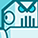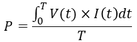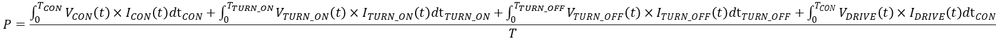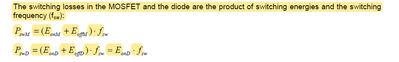Announcements

# MOSFET (Si/SiC) Forum DiscussionsLevel 1
Level 1# how to calculate power loss using mosfet product No: IAUA250N04S6N006

The mosfet I am using for my project is https://www.infineon.com/cms/en/product/power/mosfet/automotive-mosfet/iaua250n04s6n005/#!support

I am not sure how to calculate power loss.

In my project I am not using fixed frequency for mosfet On/Off, It means I have to turn on/off mosfet according to the voltage / variable value that is change all the time so the mosfet will turn on/off random manner.

for instance,  1 0 1 1 1 1 0 1 0 1 0 0 0 0 0 0 0 1 0 1 0 1 0 1 1 1 1 1 1 1 1 1 1 1 1 1 0 0 0 0 0 0 0 0 1 0 1 0 1 0 1 1 0 0 1 0 1 0 1

where; 1 for mosfet ON and 0 for mosfet OFF

1 SolutionModeratorModerator# Re: how to calculate power loss using mosfet product No: IAUA250N04S6N006

Wow, the power dissipation of MOSFET in an aperiodic behavior is a tricky problem, and I tried to explain it as follows for your reference.

As we know, the definition of instantaneous dissipation is P(t)= V(t) *I(t). This is a function of time and can change from moment to moment. For a repetitive waveform, we can find its average value over one cycle. In steady state, that average value remains constant where T=/fsw and fsw is the switching frequency):However, in your application scenario, things have changed. By definition it should be:formula_1

The total dissipation consists of a) conduction dissipation, b) turn on dissipation, c) turn off dissipation, and d) driver dissipation. Two power dissipation items are ignored:

e) Cds dissipation. This particular capacitance charges up during turn-off, and then during turn-on it dumps its stored energy inside the MOSFET.  But IAUA250N04S6N005 is only a 40V product, in low-voltage applications, this additional term may seem insignificant.

f) OFF dissipation. The leakage current through a modern semiconductor switch is almost negligible.

Now, as you mentioned, due to the random behavior of MOSFET, this formula cannot be calculated because all the time related parameters are uncertain.

Therefore, a reasonable assumption must be made. This also explains why I care about the dominant power dissipation item.

A) Constant conduction

In this case, the items b) ~f) are ignored, and the total dissipation formula can be simplified as:formula_2

B) Periodic conduction

This situation is close to the scheme you mentioned (where t=10sec), note that the cycle time is not 10s but 20s. The MOSFET is conduction within 10s and off within the next 10s.

In this case:

Pcon Equivalent to formula 2

Pturn_on = 1/6 * Vin * Id_max * tcross_on * fsw        formula 3

Pturn_off = 1/6 * Vin * Id_max * tcross_off * fsw        formula 4

Pdriver = Vdrive * Qg * fsw                                                    formula 5

Note_1: In Pturn_on/ Pturn_off calculation, the coefficient of the formula is 1/6, because I assume that you have a resistive load.  When switching an inductive load, the coefficient should be 1/2. But in reality, it seems that no application can continuously charge inductive load for 10s.

Note_2: The crossover dissipation is frequency dependent. Since the lower frequency (fsw=1/20=0.05Hz), I think the calculation result will not be much different from formula 2.

C) Statistics based calculation

In this case, formula 3~5 will no longer established, you have to use formula 1. In fact, there is no other way than to carry out a formal integration/summation procedure.

Under a specific working condition and a fixed time (for example: T=5h)time related parameters can be measured or estimated, the accuracy of the calculation results depends on the accuracy of your model. As we can see, this is not going to be easy.

Regards,

Neo

6 RepliesModeratorModerator# Re: how to calculate power loss using mosfet product No: IAUA250N04S6N006

An interesting question. When we talk about the MOS power consumption, it usually implies that this is a periodic behavior.

Since the power consumption of MOSFET is roughly composed of turn on/off loss and on loss, I want to know which part is dominant in your application.

Regards,

NeoLevel 1
Level 1# Re: how to calculate power loss using mosfet product No: IAUA250N04S6N006

Hi @Neo_Qin ,

for exp, In my project I have 10 mosfet, In which 5 are On and the Other 5 will be OFF all the time but the ON/OFF selection of mosfet is changed every time (t), lets say I check a condition every time t=10 sec then according to condition some mosfets turned On and some turned OFF, may be some mosfets are On for a long time but I cannot specify the time. I can only specify that I will check the condition every 10 seconds and then take a decesion about all mosfets.

so can I consider switching frequency  f = 1/t (where t=10sec) for power loss calculation? here still the issues is even I check condition every 10 sec , I am not sure which mosfet will be ON  and which one will be OFF? but I know that half will be on all the time and half will be off during the whole process. and the process will last for 1 to 5 hours.ModeratorModerator# Re: how to calculate power loss using mosfet product No: IAUA250N04S6N006

Wow, the power dissipation of MOSFET in an aperiodic behavior is a tricky problem, and I tried to explain it as follows for your reference.

As we know, the definition of instantaneous dissipation is P(t)= V(t) *I(t). This is a function of time and can change from moment to moment. For a repetitive waveform, we can find its average value over one cycle. In steady state, that average value remains constant where T=/fsw and fsw is the switching frequency):However, in your application scenario, things have changed. By definition it should be:formula_1

The total dissipation consists of a) conduction dissipation, b) turn on dissipation, c) turn off dissipation, and d) driver dissipation. Two power dissipation items are ignored:

e) Cds dissipation. This particular capacitance charges up during turn-off, and then during turn-on it dumps its stored energy inside the MOSFET.  But IAUA250N04S6N005 is only a 40V product, in low-voltage applications, this additional term may seem insignificant.

f) OFF dissipation. The leakage current through a modern semiconductor switch is almost negligible.

Now, as you mentioned, due to the random behavior of MOSFET, this formula cannot be calculated because all the time related parameters are uncertain.

Therefore, a reasonable assumption must be made. This also explains why I care about the dominant power dissipation item.

A) Constant conduction

In this case, the items b) ~f) are ignored, and the total dissipation formula can be simplified as:formula_2

B) Periodic conduction

This situation is close to the scheme you mentioned (where t=10sec), note that the cycle time is not 10s but 20s. The MOSFET is conduction within 10s and off within the next 10s.

In this case:

Pcon Equivalent to formula 2

Pturn_on = 1/6 * Vin * Id_max * tcross_on * fsw        formula 3

Pturn_off = 1/6 * Vin * Id_max * tcross_off * fsw        formula 4

Pdriver = Vdrive * Qg * fsw                                                    formula 5

Note_1: In Pturn_on/ Pturn_off calculation, the coefficient of the formula is 1/6, because I assume that you have a resistive load.  When switching an inductive load, the coefficient should be 1/2. But in reality, it seems that no application can continuously charge inductive load for 10s.

Note_2: The crossover dissipation is frequency dependent. Since the lower frequency (fsw=1/20=0.05Hz), I think the calculation result will not be much different from formula 2.

C) Statistics based calculation

In this case, formula 3~5 will no longer established, you have to use formula 1. In fact, there is no other way than to carry out a formal integration/summation procedure.

Under a specific working condition and a fixed time (for example: T=5h)time related parameters can be measured or estimated, the accuracy of the calculation results depends on the accuracy of your model. As we can see, this is not going to be easy.

Regards,

NeoLevel 1
Level 1# Re: how to calculate power loss using mosfet product No: IAUA250N04S6N006

Dear @Neo_Qin ,

Thank you for your detail explanation. But I am still confused, let me explain my project and why I am using IAUA250N04S6N005, then if you can help. My project is EV battery pack charging.

1. I use IAUA250N04S6N005 mosfet because I need high current and low internal resistance. I want to charge battery pack with constant current 160A where battery pack fully charged voltage is 25 volts. The mosfet I used is in-between charger and battery pack. Now I want to calculate power loss during charging in which half mosfet are on and other will be off, but in aperiodic behaviour as explained above.

2. lets say, charging time is T= 2 hours and check condition for mosfet is t=5sec (so mosfet total period will be t=10sec, as you explained).

3. Condition loss as explained in the documents  https://community.infineon.com/t5/MOSFET-Si-SiC/MOSFET-Switching-Losses/td-p/321512 MOSFET Power Losses Calculation Using the Data-Sheet Parameters.

Pcon=Rdson * (Id)^2  =  0.64*10^-3   *   160^2

4. Switching loss calculation is very complicated, as given in MOSFET Power Losses Calculation Using the Data-Sheet Parameters.I am not sure yet, how to calculate Eonm and Eoffm in above formula, but even if I calculate it, the main issue is how to define fsw ? is it ok to assume fsw=1/t (t=10sec).ModeratorModerator# Re: how to calculate power loss using mosfet product No: IAUA250N04S6N006

Integration is the only way to calculate E_cross. If we plot every Vd(t)*Id(t) point out during the crossover, we may get an irregular curve, area enclosed by this curve and the time axis is the energy lost during the transition.

The attempt to get the area of an irregularly closed plane is not simple. However, when the load of the MOSFET is purely inductive or resistive, there are mature simplified schemes. Basically, every manufacturer provides similar application note, the difference is only in the literary style.

Unfortunately, there is almost no literature to analyze the switching behavior of MOSFETs under capacitive load (maybe my retrieval ability is insufficient).  And that's exactly what you need right now, because battery can simply be equivalent to a capacitor. In this case, I recommend performing a bench test to get the power dissipation data during the transition. Some oscilloscopes have commercially power analysis software that may help, you can also export data to Excel for summation calculation.

Why is there almost no literature analyzing capacitive loads? Perhaps because in the real world, there is almost no fast & periodic switching capacitor behavior (low power charge pump converter may be an exception). This also means that people do not care about the power loss during transition, it can be reasonably ignored.

In your application, averaging the sum of all crossover-losses (note that 2h=7200s is a large denominator value) or assuming a very low frequency periodic waveform, seems meaningless. With Equation Pd=Rds*square (Id), you can can already get average power dissipation with acceptable accuracy. The temperature rise test can also be carried out to prove that all MOSFETs operate within a safe temperature range. This is simple and reliable.

But is it really unnecessary to analyze crossover process? The answer is No.

During the turn-on transition, a large inrush current is expected (Corresponding the peak power dissipation), especially when the capacitance value is very large (I=C*dV/dt). This situation must be handled carefully, because MOSFET works in the linear region at this time. The SOA analysis is performed to verify that MOSFET will not be damaged. The turn-off transition process is the same.

Another noteworthy issue is the current sharing of MOSFETs. Since the conduction time may be short, which not enough to bring multiple paralleled MOSFETs to reach the thermal balance, there is always one specific MOS that will be subjected to a largest current at the moment of switching. This also means that it is easier to be damaged.

An Infineon application note is shown as below, where switching capacitive loads is mentioned.

https://www.infineon.com/dgdl/Infineon-ANPS061E-AN-v01_00-EN.pdf?fileId=5546d4625b62cd8a015baa1aac28...

Regards,

NeoModeratorModerator# Re: how to calculate power loss using mosfet product No: IAUA250N04S6N006